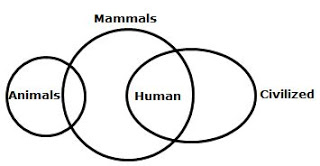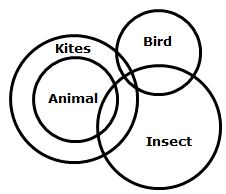# Reasoning Quiz for Bank ExamsReasoning Quiz
Welcome to Mentor for Bank Exams. Here I am sharing questions that are much helpful in the preparation for upcoming bank exams like IBPS PO, IBPS Clerks, IBPS RRB and other Insurance exams. Kindly make use of these!!!
Directions (1-5): In each question below are given two statements followed by two conclusions numbered I and II. You have to take the two given statements to be true even if they seem to be at variance with commonly known facts. Read the conclusions and then decide which of the given conclusions logically follows from the given statements disregarding commonly known facts.
a) If only conclusion I follows
b) If only conclusion II follows
c) If either I or II follows
d) If neither I nor II follows
e) If both I and II follow
1. Statements: All humans are civilized.
Some animals are mammals.
All humans are mammals.
Conclusion:
I. All civilized are mammals.
II. Some animals are civilized.
2. Statements: Smita is a girl.
Some girls are beautiful.
All girls are student.
Conclusion:
I. Smita is an student.
II. Some students are beautiful.
3. Statements: All rivers are streams.
Some streams are wells.
No well is sea.
Conclusion:
I. Some rivers are wells.
II. Some seas are not stream.
4. Statements: Some kites are Bird.
All animals are kites.
Some animals are insects.
Conclusion:
I. No bird is insect.
II. Some insect are kites.
5. Statements: Some doctors are professional.
Raj is a professional.
Raj is not young.
Conclusion:
I. Some doctors are young.
II. Some young doctors are not professional.
Directions (6 – 10): A coded statement is followed by two conclusions. Decode the statement and conclusion
The symbols have to be decoded as follows:
A @ B means A is smaller than B.
A # B means A is greater or equal to B.
A \$ B means A is greater than B.
A % B means A is equal to B.
A & B means A is shorter or equal to B.
a) If only conclusion I is true
b) If only conclusion II is true
c) If conclusion I or II is true
d) If neither conclusion I nor II is true
e) If both conclusions are true
6. Statement: V @ W, W # L, L\$Y
Inference: I. L \$ V
II. V @ Y
7. Statement: G # H, H % O, O @ F
Inference: I. G \$ F
II. G @ F
8. Statement: D & K, K \$ P, P # Q
Inference: I. K # Q
II. D & Q
9. Statement: S \$ R,R % T, T @ V
Inference: I. V @ S
II. V @ R
10. Statement: N @ L, L% P, P & H
Inference: I. L & N
II. H \$ N
Direction (11 – 15): Study the following information carefully and answer the questions followed:
In Andhra Layola college, Vijayawada, there are seminars on seven different subjects: Physics, Chemistry, Math, Biology, History, Hindi and English from Monday to Sunday, not necessary in the same order. The duration of the lectures are 1 hour, 1.5 hour or 2 hours. History is the 2nd last lecture to be taught and has a duration of 1 hour. The lecture on physics is followed by the lecture on math which is of 1.5 hours. The lecture on Biology is of two hours but it is neither on Monday nor on Sunday. The lecture on Hindi is on Thursday and it is neither of 2 hours or 1.5 hour. The lectures held on Monday and Sunday are of 2 hours each. The English lecture is not of 2 hours and is just after a 1 hour lecture.
11. Which is the last lecture to be held and of what duration?
a) English 2h
b) Physics 2h
c) Chemistry 2h
d) Biology 2h
e) Can’t be determined
12. Which lecture is held on Tuesday?
a) Biology
b) English
c) Math
d) Physics
e) Can’t be determined
13. What is the duration of the English lecture?
a) 1 hour
b) 2 hour
c) 1.5 hour
d) 1 hour or 2 hour
e) None of these
14. What are the total teaching hours between the lectures on Math and English?
a) 3 hours
b) 2 hours
c) 3.5 hours
d) 2.5 hours
e) Can’t be determined
15. Which of the following options is correct?
a) The duration of lecture on English is less than the duration of lecture on Chemistry
b) The lecture on Biology is held immediately after the lecture on Math.
c) Lectures which are held on Thursday and Saturday are of the same duration.
d) The duration of Math lecture is more than the duration of at least two lectures.
e) all of the above
1)d   2)e   3)d   4)b   5)d   6)a   7)b   8)d   9)d   10)e   11)c   12)c   13)e   14)a   15)e
Solutions:
1. D)2. E)3. D)4. B)5. D)6. A) V < W, W L, L > Y
or, W > V, W L, L > Y
or, W =L > V, W > L > V, W = L > Y
there is no clear indication between V and Y.
7. B) G H, H = O, O < F
or, G H, H = O, F > O
or, G > H, G = H = O, F > G = H = O
8. D) D K, K > P, P Q
or K D, K > P, P Q
or, K > D, K = D > P, P Q
or, K > P Q, K = D > P Q
thus, I and II both are not true
9. D) S > R, R = T, T < V
or, S > R =T, V > R = T
thus, I and II both are not true
10. E) N < L, L =P, P H
or, L>N, L = P-, HP
or, L = P > N, H P = L
(11 – 15):
 Day Monday Tuesday Wednesday Thursday Friday Saturday Sunday Time 2hrs 1.5hrs 2hrs 1hr 1hr or 1.5hrs 1hr 2hr Subject Physics Maths Biology Hindi English History Chemistry
11. C)
12. C)
13. E)
14. A)
15. E)